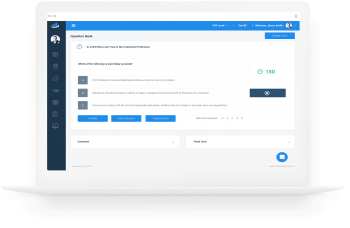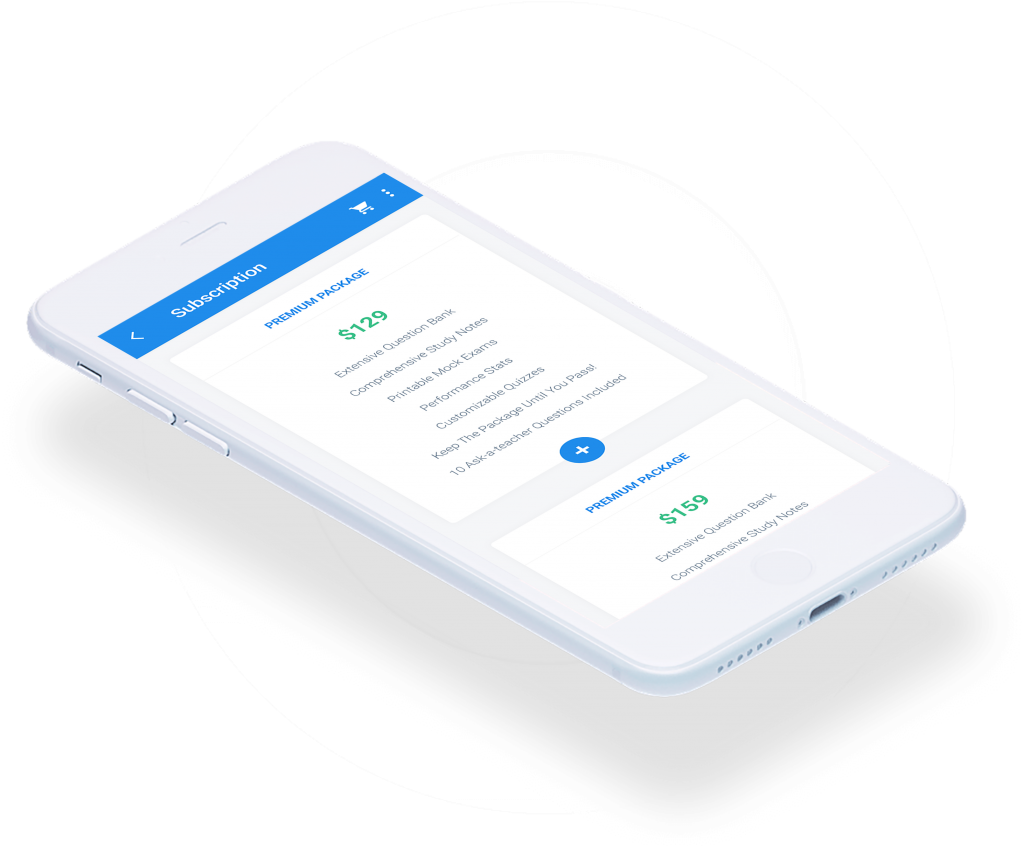Limited Time Offer: Save 10% on all 2021 and 2022 Premium Study Packages with promo code: BLOG10    Select your Premium Package »

No-Arbitrage Values of Options

Valuing European Options A European option is an option that can only be exercised at expiry. Consider a stock with an initial price of \$70 and a risk-free rate of 1% per year. The asset price can move up by…

Binomial Option Valuation Model

Contingent Claims A contingent claim is a derivative contract that gives the owner the right but not the obligation to receive a future payoff that depends on the value of the underlying asset. Call and put options are examples of…

Implied Volatility

We have seen that both the BSM model and Black model require the parameter, $$\sigma$$ which is the volatility of the underlying asset price. However, future volatility cannot be observed directly from the market but rather estimated. One way of…

Role of Gamma Risk in Options Trading

Gamma measures the risk that remains once the portfolio is delta neutral (non-linearity risk). The BSM model assumes that share prices change continuously with time. In reality, stock prices do not move continuously. Instead, they often jump, and this creates…

Delta Hedging

Delta hedging involves adding up the deltas of the individual assets and options making up a portfolio. A delta hedged portfolio is one for which the weighted sums of deltas of individual assets is zero. A position with a zero…

Option Greeks

The Greeks are a group of mathematical derivatives applied to help manage or understand portfolio risks. They include delta, gamma, Theta, Vega, and rho. Delta Delta is the rate of change of the option’s price with respect to a given…

Black Model Valuation of Interest Rate Options and Swaptions

Interest Rate Options The underlying instrument in an interest rate swap is a reference interest rate. Reference rates include the Fed funds rate, Libor, and the rate on benchmark US Treasuries. Interest rate options are, therefore, options on forward rate…

Black Option Valuation Model

The black option valuation model is a modified version of the BSM model used for options on underlying securities that are costless to carry, including options on futures and forward contracts. Similar to the BSM model, the black model assumes…

Valuation of Equities and Currencies using the Black-Scholes Model

Some underlying instruments have carry benefits. These benefits include dividends for stock options, foreign interest rates for currency options, and coupon payments for bond options. The BSM model should be adjusted to incorporate carry benefits in the option value. Let…

Components of the BSM Model

The BSM model for pricing options on a non-dividend-paying stock is given by: European call $$c_{0}=S_{0}N(d_{1})-e^{-rT}KN(d_{2})$$ European Put $$p_{o}=e^{-rT}KN(-d_{2})-S_{0}N(-d_{1})$$ Where:  $$d_{1}=\frac{ln\bigg(\frac{S}{K}\bigg)+\bigg(r+\frac{1}{2}\sigma^{2}\bigg)T}{\sigma\sqrt{T}}$$ $$d_{2}=d_{1}-\sigma\sqrt{T}$$ $$N(x)=$$ standard normal cumulative distribution function $$N(–x) = 1 – N(x)$$ BSM Model has the following variables: $$T=$$…

Preparation Platform for the Three Levels of the CFA® ExamView More
Recent Posts
FRM® Study PlatformView More
Video Series – Level I of the CFA® Exam